Electrical Tester online
March 2016
Sweep frequency response analysis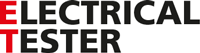# Sweep frequency response analysis

01 March 2016

Sweep Frequency Response Analysis (SFRA) is a powerful and sensitive method for evaluating the mechanical integrity of core, windings and clamping structures within power transformers. Its basis is the measurement of the transformers’ electrical transfer functions over a wide frequency range. The goal of SFRA is to test if physical displacements in the active part of the transformer have occurred due to aging or after a particular event such as short circuit fault and transportation. The test results are compared with the reference so that a wide range of fault types can be detected, including:

• Core movements
• Faulty core grounds
• Winding deformations
• Winding displacements
• Partial winding collapse
• Hoop buckling
• Broken clamping structures
• Shorted turns and open windings
• Etc.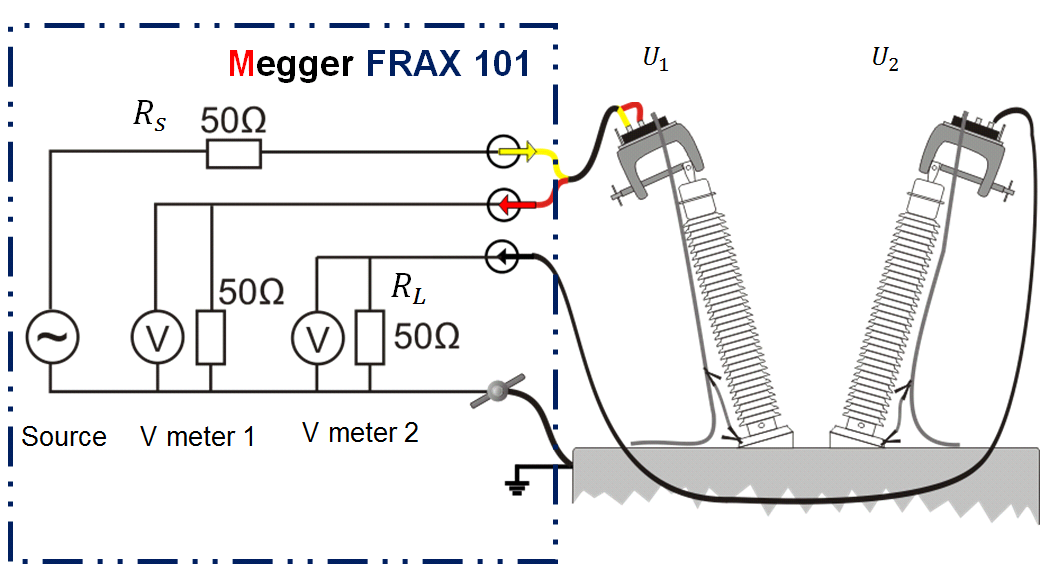Figure 1 – SFRA test setup and schematic diagram of the testing instrument

Measured parameters and result interpretations

The SFRA method involves injecting a signal at one terminal of the transformer and measuring the resultant signal at another terminal as shown in Figure 1. The measured parameters are the applied terminal voltage   and the resultant   at another terminal. The result is shown in magnitude (dB) and phase angle (°) which are expressed as:The voltage  is the voltage across a 50 Ohm resistor, which is proportional to the current flowing through the transformer winding. Therefore the magnitude represents the admittance information of the transformer. The test results are usually shown as the magnitude plotted against frequency on a logarithmic scale. Three phases are measured respectively and then compared with each other as shown in Figure 2. Three identical traces indicates good condition while large deviations may mean the existence of a problem such as deformation.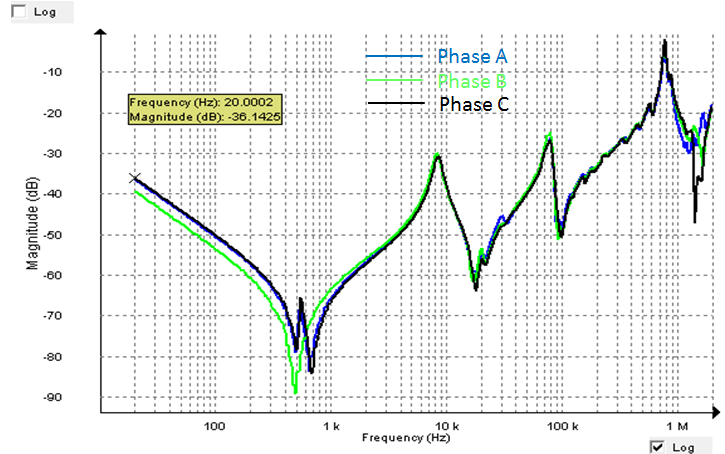(a)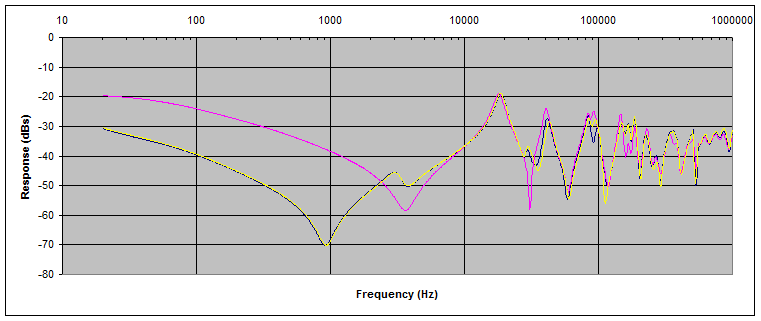(b)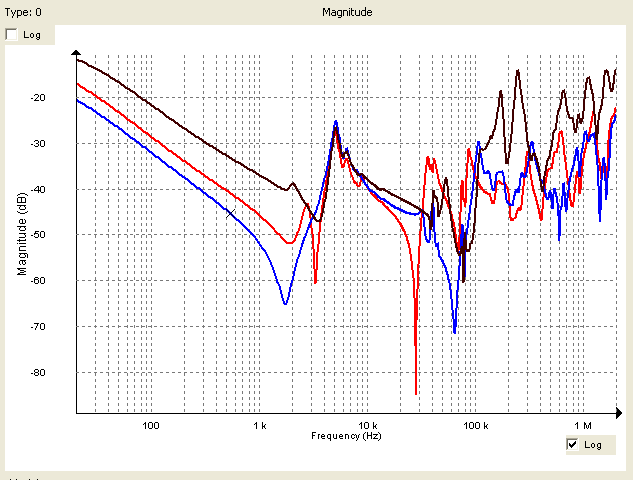(c)

Figure 2 – the transformer (a) in good condition (b) with shorted turns in one phase (c) in very bad condition

For a three-phase transformer with two windings, a recommended minimum of nine tests are performed, as shown in Figure 3.

• Measure the three phase responses from HV side with LV side short-circuited
• Measure the three phase responses from HV side with LV side open-circuited
• Measure the three phase responses from LV side with HV side open-circuited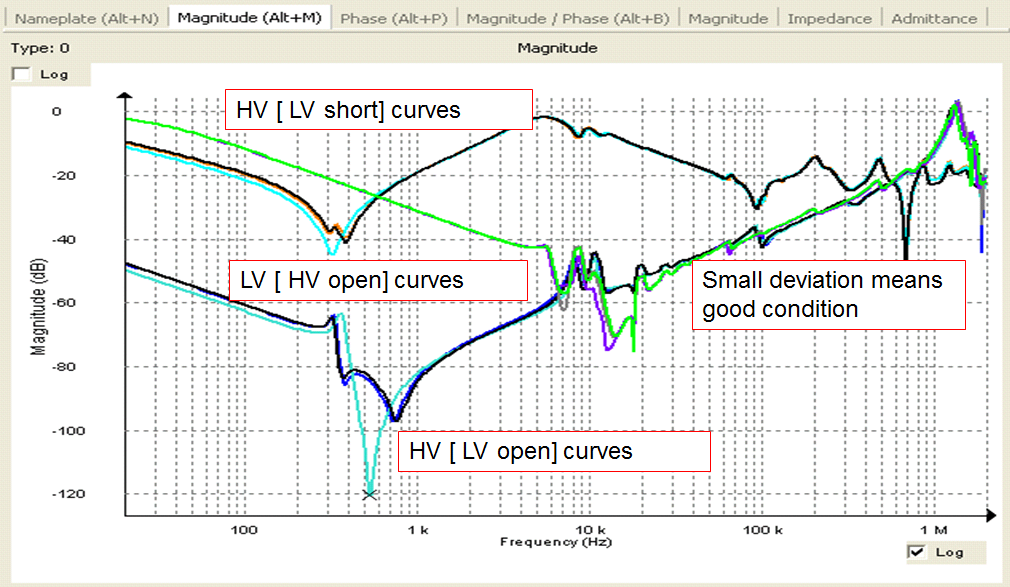Figure 3 – Typical SFRA result of a three-phase transformer

The “open” measures the excitation impedance of the transformer while the “short” measures the short-circuit impedance of the transformer. It is important to point out that for the “open” measurements, the phase B trace often deviates from phase A and C traces at low frequencies due to the core structure. This should not be treated as a defect. I As the frequency increases, the inductance introduced by the core starts to decrease and then disappears. “Open” and “short” measurements give the same results at high frequencies.

In addition to comparison between phases, there are two more comparative methods:

• Time-based comparison: SFRA results are compared to previous results for the same unit. This is the easiest and most effective method by which to discern a problem.

• Type-based comparison: SFRA of one transformer is compared to a similar type of transformer. A sister transformer is considered one having the same nameplate data and usually the same manufacturer.

Different faults can be identified from the change of the SFRA curve in certain frequency ranges. The low-frequency curve shape is determined by the magnetic circuit of a transformer. So core problems, shorted turns and broken strands change this shape. The bulk winding capacity is around a few nanofarads and it starts to affect the SFRA curve from a few kHz. Winding disc capacitance is quite small and starts to play a role in the highest frequency range. Table 1 may help test engineers to identify the specific problem based on the curve differences in certain frequency ranges.

Table 1 – Mechanical faults and their related frequency band for reference 

Frequency sub-band

Component

Possible failure

< 2 kHz

Core
Winding inductance

Core deformation, core ground problem, shorted turns, open circuits

2 kHz to 20 kHz

Bulk Component

Bulk winding movement

20 kHz to 400 kHz

Main winding

Deformation within the main or tap windings

400 kHz to 2 MHz

Main winding, tap winding and internal leads

Movement of the main and tap windings, ground impedance variations

After de-tanking a transformer, the deformation shown in Figure 4 gave the frequency response shown in Figure 5. It can be seen that the phase B curve changes its pattern after the fault. The deviation is between 500 Hz to 500 kHz, and it could be concluded that the core is all right but the winding is deformed. The difference is most obvious from 1 to 10 kHz while the low frequency part is all right. According to Table 3, the problem is most likely bulk winding deformation.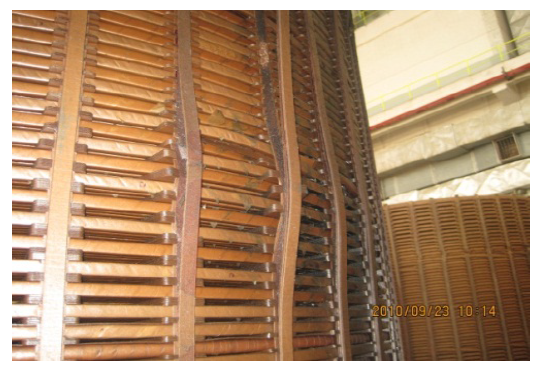Figure 4 – Transformer winding buckling fault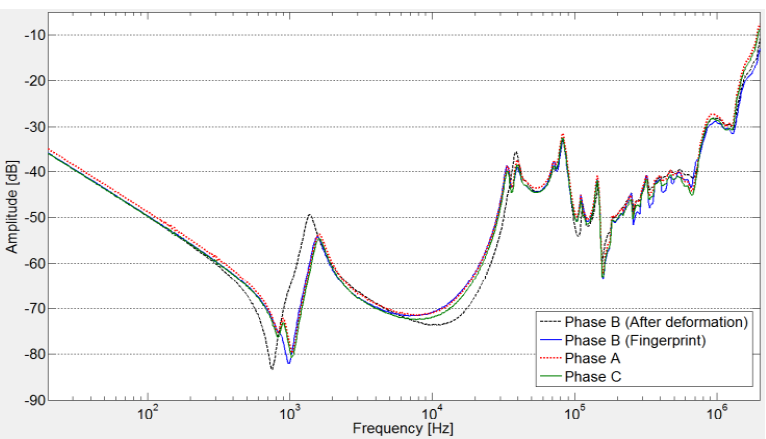Figure 5 – Sweep Frequency Responses of the transformer that has the winding buckling fault

Transformer Modelling
To understand why SFRA testing is able to detect so many mechanical faults that are hard to detect by other methods, the equivalent circuit model of the transformer under test needs to be analysed. The model discussed in this article is valid for single-phase transformers.

At low frequencies (< 1 kHz), the equivalent circuit model of transformers is shown in Figure 6. It consists of an ideal transformer, plus the excitation impedance and leakage impedances of HV and LV windings.  When the SFRA signal is injected from the HV winding with LV winding open, it is actually measuring the excitation impedance. The impedance increases linearly with test frequency and thus forms a straight line on a logarithm scale.

As the frequency increases, the capacitive effect starts to appear and the core inductance becomes less dominant. The first resonance point is the resonance of the inductor, determined by winding and core, and the capacitor, constructed by winding and ground (core and tank).

At the highest frequency, the resonance is due to the self-inductance of conductors (nH/m) and the capacitor of winding discs/turns. The high frequency equivalent circuit is shown in Figure 7. The number represents winding turns. Each turn consists of self-inductance, mutual inductance and resistance. In addition to that, there are turn-to-turn capacitors (an ideal capacitor and the loss component) and turn-to-ground capacitors.

Power transformer internal faults can produce shifts in some regions of the frequency response curve, and these shifts can be identified by visual inspection.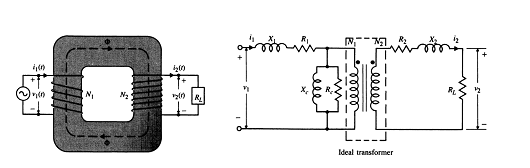Figure 6 – Single-phase transformer model at low frequencies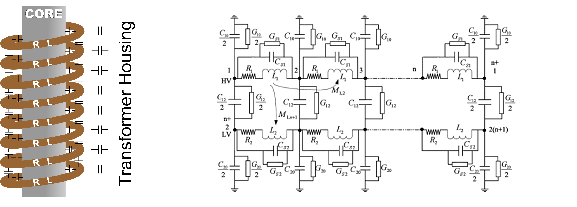Figure 7 – Single-phase transformer model at 1 MHz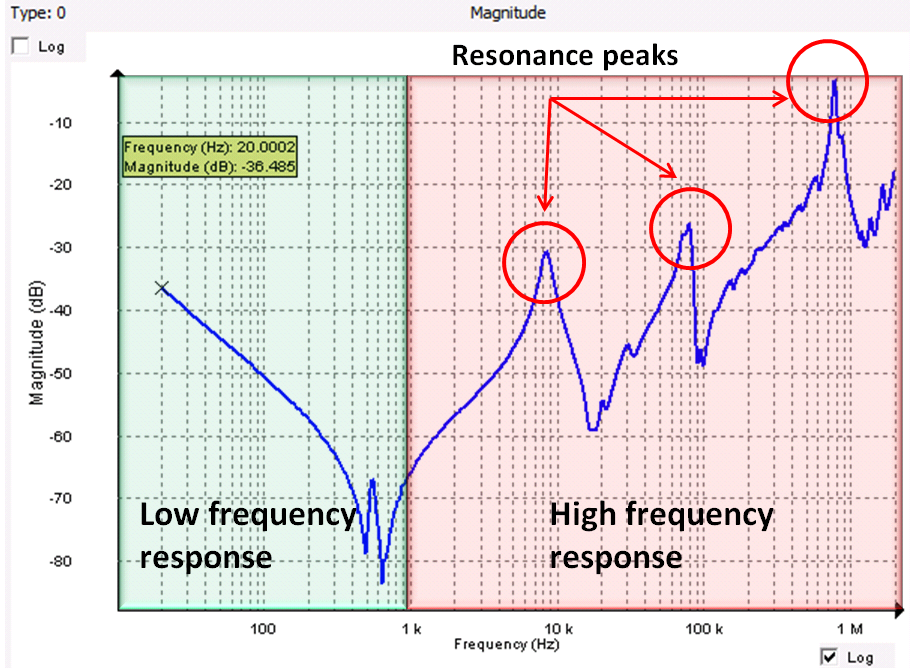Figure 8 – Characteristics of an SFRA curve

Good practices in SFRA testing
SFRA is a low voltage ( 10V), non-destructive test method. Good practices must be followed to guarantee the quality of the results.

Apply consistent test voltage
It has been proven that the low frequency response is mainly determined by the magnetic properties of the transformer. The permeability of the magnetic core material is usually assumed to be constant but in practice it is not. It varies with the applied voltage. The frequency response is measured at quite a low voltage – only around 10V. The permeability of the core changes rapidly at this voltage level.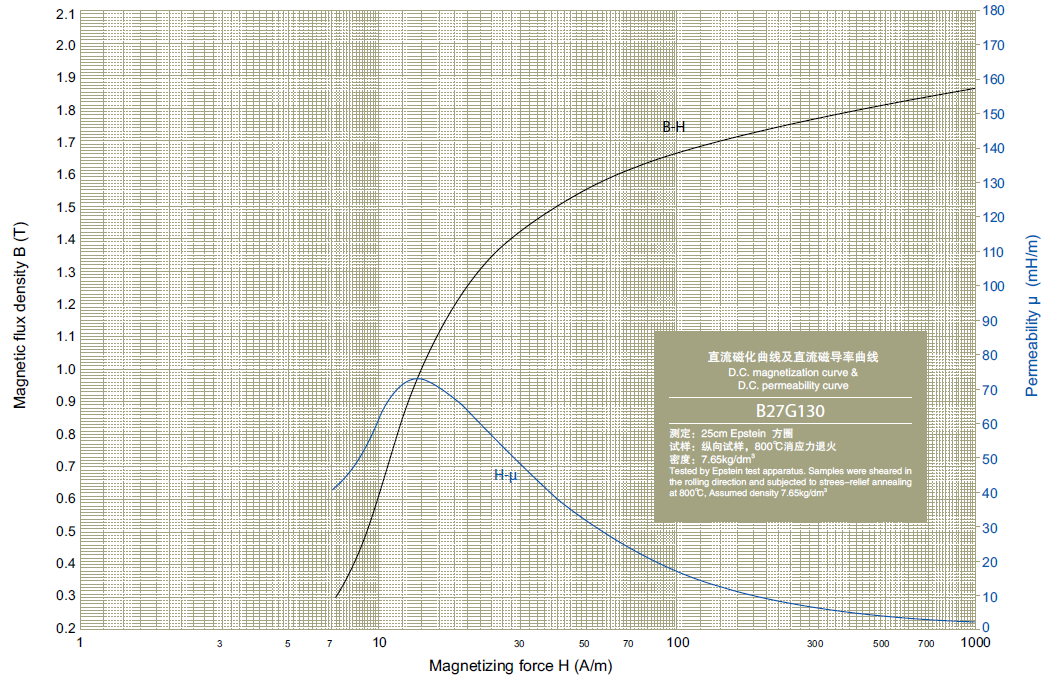Figure 9 – B-H curve and μ-H curve of transformer core material (B27G130 silicon-iron )

The core permeability varies with the applied voltage, which makes the SFRA responses at low frequencies voltage dependent. Consistent voltage is, therefore, very important for SFRA testing. A test instrument with variable output voltage is suggested so that the test can be performed at the same voltage as the reference curve.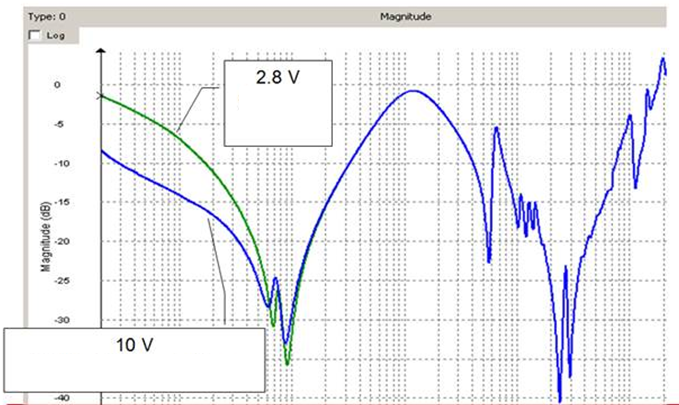Figure 10 – SFRA results with different applied voltages

Keep an eye on the tap changer position
The tap changer positions must be the same as used for benchmark tests, otherwise the frequency responses will differ and may not be compared. If the maximum tap is chosen then the whole tap changer is examined by the test. This applies for both DETC and OLTC. Typically the test will be carried out at full winding position and neutral.

Demagnetization first
The residual flux has influence on the SFRA results. After the winding resistance test, the starting point goes from point 0 to point 1 or point 2 on the hysteresis curve – see Figure 11. Since the residual flux changes the core inductance significantly, it is recommended to do the SFRA test before any other test or immediately after demagnetization, which makes the start point go back to point 0.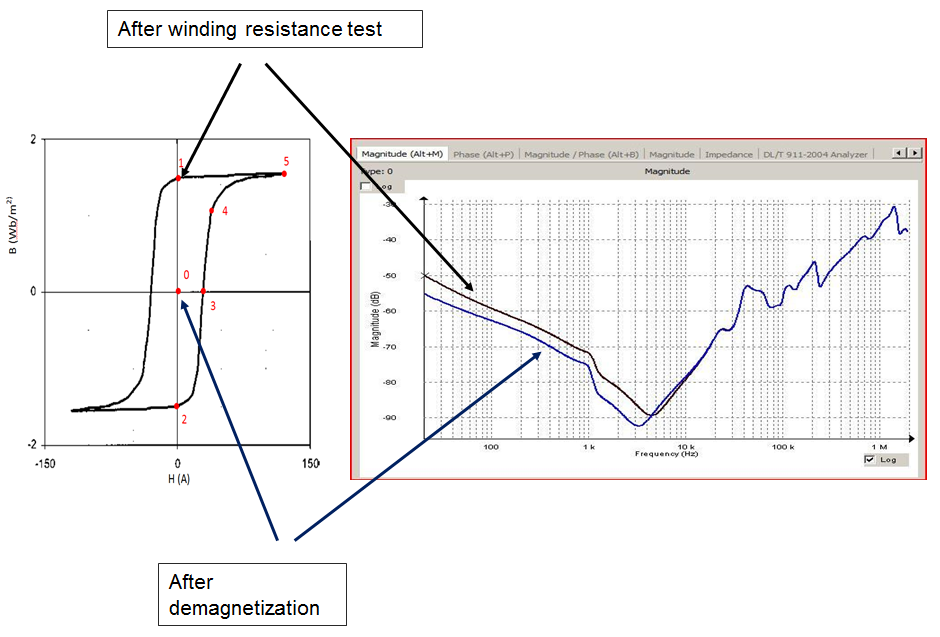Figure 11 – SFRA Responses obtained before and after demagnetization

The high frequency part of the response is very sensitive to electromagnetic interference, so the test system should be noise resistant. One widely used approach is the use of the coaxial cable. The centre conductor of the cable is connected to the transformer terminal while the outer conductor is connected to the instrument chassis, which should be grounded. This practice prevents the centre conductor from picking up noise.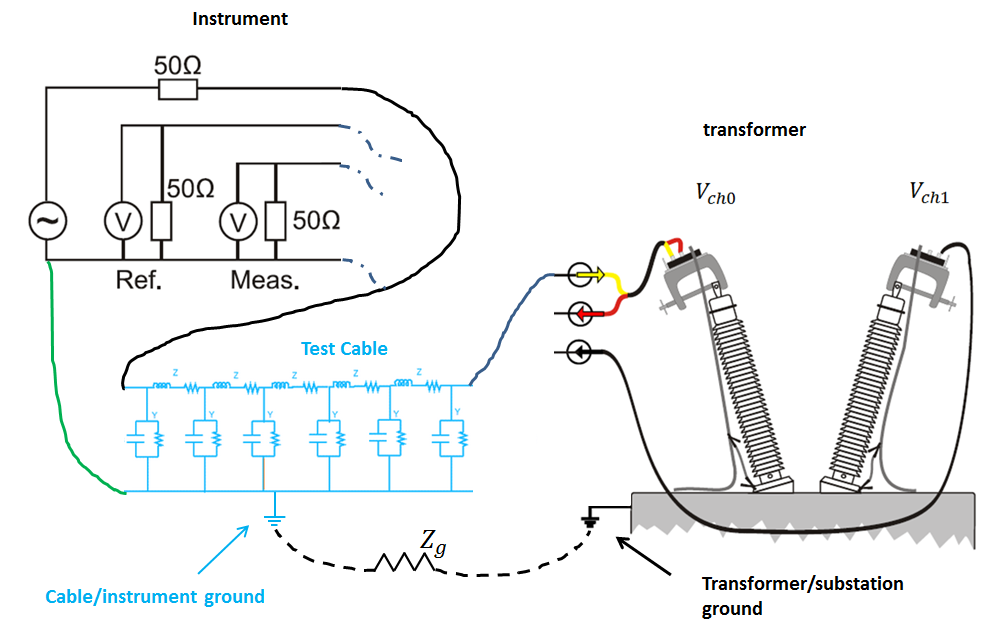Figure 12 – Two ground systems

It is quite common that the transformer ground (substation ground) is not the same ground as the instrument ground. In such cases, the current and voltage between the transformer ground and the instrument ground may adversely affect the test result. To improve repeatability, it is necessary to connect the cable ground (shield) and transformer ground together with the shortest possible flat braids as shown in Figure 1.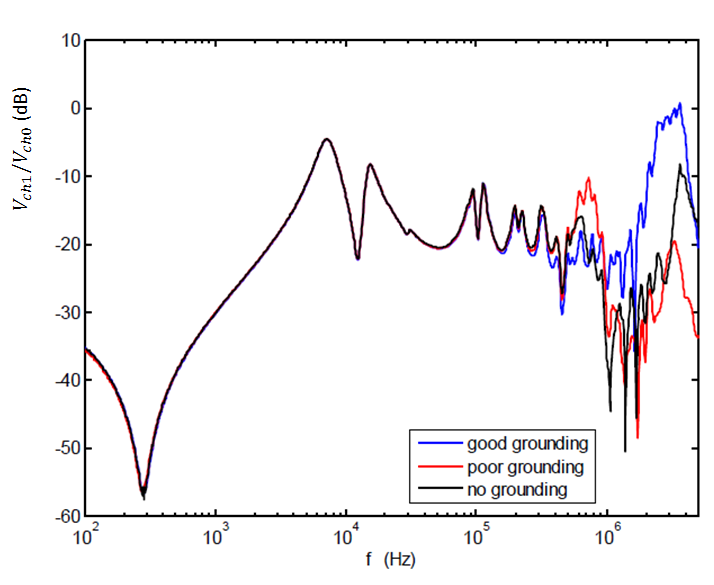Figure 13 – Results obtained with different grounding practices

Conclusion
SFRA is an invaluable test technique sensitive to problems that would otherwise be difficult to detect without dismantling the transformer – a costly and very inconvenient exercise. To acquire the most representative results, however, a high quality test set of proven performance must be used, good practices must be observed during testing and the results must be interpreted with care, following the guidelines given in this article.

Megger's selection of sweep frequency response analysers can be seen by clicking here.

References
 J.C. Gonzales and E.E. Mombello, “Diagnosis of Power Transformers through Frequency Response Analysis by Poles and Zeros Shifts Identification,” Transmission and Distribution: Latin America Conference and Exposition (T&D-LA), 2012 Sixth IEEE/PES
 M. Bagheri, M. S. Naderi, T. Balckburn and T. Phung, “FRA vs. Short Circuit Impedance Measurement in Detection of Mechanical Defects within Large Power Transformer,” Conference Record of the 2012 IEEE International Symposium on Electrical Insulation (ISEI)
 N. Abeywickrama, Y. Serdyuk and S. Gubanski, “High-Frequency Modeling of Power Transformers for Use In Frequency Response Analysis,” IEEE Trans. on Power Delivery, Vol. 23, No.4, 2008
 Technical Manual of Baosteel GO silicon Steel Products, 2008